# OLD ARTICLE – RMS or Root Mean Square Value of AC Signal

Why rms values are used in AC system?
What does an average and rms value mean?
Why all the ratings of AC systems are in rms not in average value?
What is the difference between rms and average value?

These are the questions which come in our minds every time when we are dealing with AC circuits.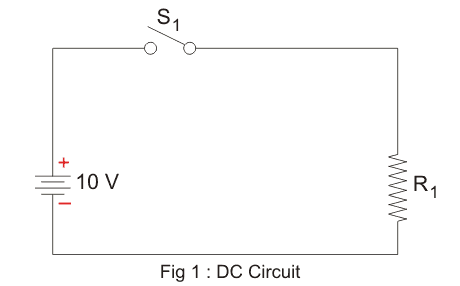Suppose, we have a simple DC circuit (figure – 1) and we want to replicate it in an AC circuit. We got every thing same, except supply voltage which is now to be an AC supply voltage. Now, the question is what should be the value of AC supply voltage so that our circuit works exactly same as that of DC.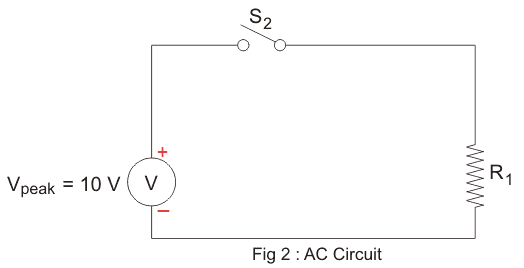Let us put same value of AC supply voltage (AC Vpeak = 10 volt) which is in our DC circuit. By doing that we can see (figure 3) for a half cycle how the AC voltage signal is not covering up the whole area (blue area) of constant DC voltage, which means our AC signal can not supply the same amount of power as our DC supply.

Which means we must increase the AC voltage to cover the same area and see if it is supplying the same amount of power or not.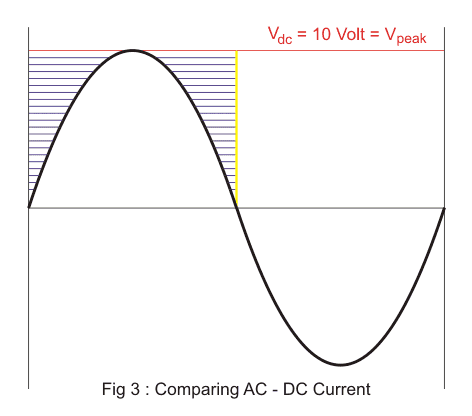We found that (figure 4) by increasing the peak voltage Vpeak up to (π/2) times of DC supply voltage we can actually cover the whole area of DC in AC. When the AC voltage signal completely represents the DC voltage signal then that value of DC signal is called the average value of AC signal.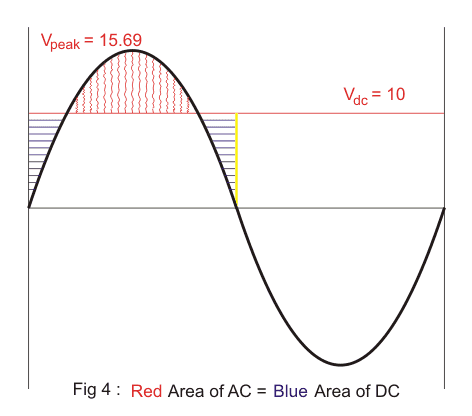Now our AC voltage should supply the same amount of power. But when we switched-on the supply surprisingly, we found that AC voltage is supplying more power than the DC. Because an average value of AC supplies same amount of charges but not the same amount of power. So, to get same amount of power from our AC supply we must decrease our AC supply voltage.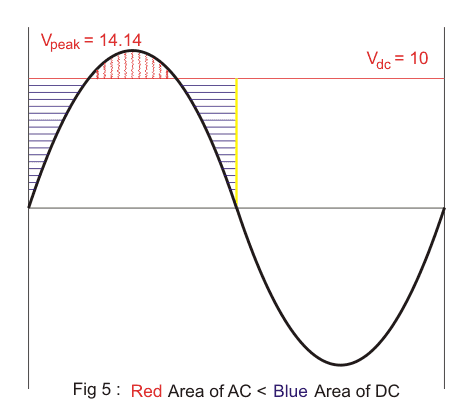We found that by decreasing the peak voltage Vpeak up to √2 times DC voltage we get same amount of power flowing in both the circuits. When the AC voltage signal supply same amount of power as in DC then that value of DC voltage is called root mean square or rms value of AC.
We are always concerned about how much power is flowing through our circuits irrespective of how much electrons are needed to supply that power and that is the reason why we always use the rms value of AC supply instead of average value everywhere in AC system.

Conclusion
Average value of an AC current represent the equal amount of charges in DC current.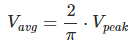RMS value of an AC current represent the equal amount of power in DC current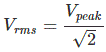AC current takes less amount of charges to supply the same amount of DC power.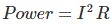Want To Learn Faster? 🎓
Get electrical articles delivered to your inbox every week.
No credit card required—it’s 100% free.